Next: MHD Dynamo Theory Up: Magnetohydrodynamic Fluids Previous: Interplanetary Magnetic Field

# Mass and Angular Momentum Loss

Since the Sun is the best observed of any star, it is interesting to ask what impact the solar wind has as far as solar, and stellar, evolution are concerned. The most obvious question is whether the mass loss due to the wind is significant, or not. Using typical measured values (i.e., a typical solar wind velocity and particle density at 1 AU of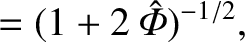and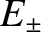, respectively), the Sun is apparently losing mass at a rate of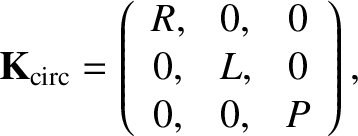per year, implying a time-scale for significant mass loss of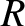years, or some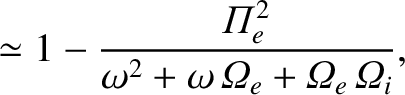times longer than the estimated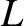year age of the Sun. Clearly, the mass carried off by the solar wind has a negligible effect on the Sun's evolution. Note, however, that many other stars in the Galaxy exhibit significant mass loss via stellar winds. This is particularly the case for late-type stars.

Let us now consider the angular momentum carried off by the solar wind. Angular momentum loss is a crucially important topic in astrophysics, since only by losing angular momentum can large, diffuse objects, such as interstellar gas clouds, collapse under the influence of gravity to produce small, compact objects, such as stars and proto-stars. Magnetic fields generally play a crucial role in angular momentum loss. This is certainly the case for the solar wind, where the solar magnetic field enforces co-rotation with the Sun out to the Alfvén radius,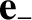. Thus, the angular momentum carried away by a particle of mass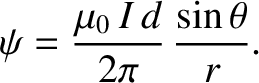is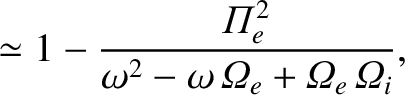, rather than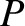. The angular momentum loss time-scale is, therefore, shorter than the mass loss time-scale by a factor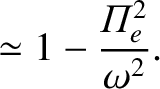, making the angular momentum loss time-scale comparable to the solar lifetime. It is clear that magnetized stellar winds represent a very important vehicle for angular momentum loss in the Universe. Let us investigate angular momentum loss via stellar winds in more detail.

Under the assumption of spherical symmetry and steady flow, the azimuthal momentum evolution equation for the solar wind, taking into account the influence of the interplanetary magnetic field, is written(770)

The constancy of the mass flux [see Eq. (744)] and thedependence of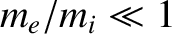[see Eq. (763)] permit the immediate integration of the above equation to give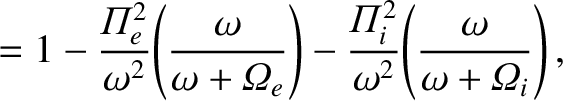(771)

where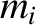is the angular momentum per unit mass carried off by the solar wind. In the presence of an azimuthal wind velocity, the magnetic field and velocity components are related by an expression similar to Eq. (761):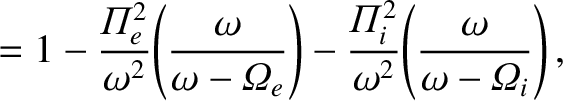(772)

The fundamental physics assumption underlying the above expression is the absence of an electric field in the frame of reference co-rotating with the Sun. Using Eq. (772) to eliminate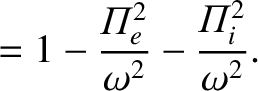from Eq. (771), we obtain (in the ecliptic plane, where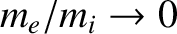)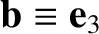(773)

where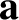(774)

is the radial Alfvén Mach number. The radial Alfvén Mach number is small near the base of the corona, and about 10 at 1 AU: it passes through unity at the Alfvén radius,, which is about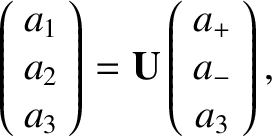AU from the Sun. The zero denominator on the right-hand side of Eq. (773) at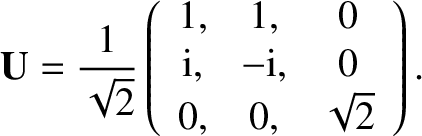implies that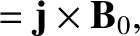is finite and continuous only if the numerator is also zero at the Alfvén radius. This condition then determines the angular momentum content of the outflow via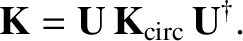(775)

Note that the angular momentum carried off by the solar wind is indeed equivalent to that which would be carried off were coronal plasma to co-rotate with the Sun out to the Alfvén radius, and subsequently outflow at constant angular velocity. Of course, the solar wind does not actually rotate rigidly with the Sun in the region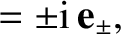: much of the angular momentum in this region is carried in the form of electromagnetic stresses.

It is easily demonstrated that the quantity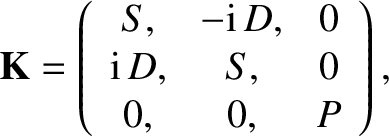is a constant, and can, therefore, be evaluated atto give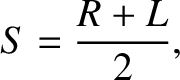(776)

where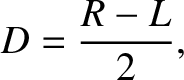. Equations (773), (775), and (776) can be combined to give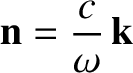(777)

In the limit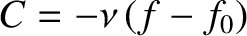, we have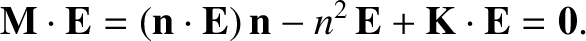, so the above expression yields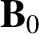(778)

at large distances from the Sun. Recall, from Sect. 5.7, that if the coronal plasma were to simply co-rotate with the Sun out to, and experience no torque beyond this radius, then we would expect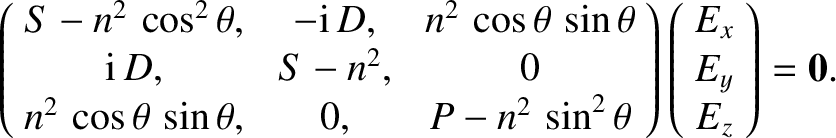(779)

at large distances from the Sun. The difference between the above two expressions is the factor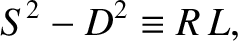, which is a correction for the angular momentum retained by the magnetic field at large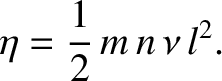.

The analysis presented above was first incorporated into a quantitative coronal expansion model by Weber and Davis.The model of Weber and Davis is very complicated. For instance, the solar wind is required to flow smoothly through no less than three critical points. These are associated with the sound speed (as in Parker's original model), the radial Alfvén speed,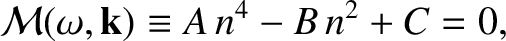, (as described above), and the total Alfvén speed,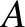. Nevertheless, the simplified analysis outlined above captures most of the essential features of the outflow. For instance, Fig. 20 shows a comparison between the large-asymptotic form for the azimuthal flow velocity predicted above [see Eq. (778)] and that calculated by Weber and Davis, showing the close agreement between the two.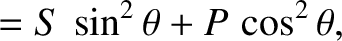Next: MHD Dynamo Theory Up: Magnetohydrodynamic Fluids Previous: Interplanetary Magnetic Field
Richard Fitzpatrick 2011-03-31